Question

# Given the following code fragment public class Point { public int x; // Because these are...

Given the following code fragment

public class Point {

public int x; // Because these are public, you can access them public int y; // directly without getters and setters

```    };
public class Rectangle {
```
```        private Point ll; // the lower left corner of the rectangle
private Point ur; // the upper right corner of the rectangle
public Point getLLPoint() {return ll;}
public Point getURPoint() {return ur;}
```

}

1. (a) Write a method equals for the Rectangle class that takes a Rectangle as a parameter and returns true if two rectangles have the same area, and false otherwise

2. (b) Write a method manhattanDistance for the Point class that takes a Point object and calculates the distance between the current Point and the the given other Point assuming travel only along horizontal and vertical lines (e.g. between the manhattan distance between (0,0) and (1,1) would be 2)

public boolean equal_area(Rectangle r1,Rectangle r2){
int r1_length = r1.getURPoint().x - r1.getLLPoint().x;
int r1_breadth = r1.getLLPoint().y - r1.getURPoint().y;
int r2_length = r2.getURPoint().x - r2.getLLPoint().x;
int r2_breadth = r2.getLLPoint().y - r2.getURPoint().y;
if (r1_area == r2_area)
return true;
return false;
}
public int manhattanDistance(Point p){
return abs(p.x - x) + abs(p.y - y);
// abs is absolute value
}

#### Earn Coins

Coins can be redeemed for fabulous gifts.

Similar Homework Help Questions
• ### public class Point f private int x; private int y; public Point(int x, int y) this.x...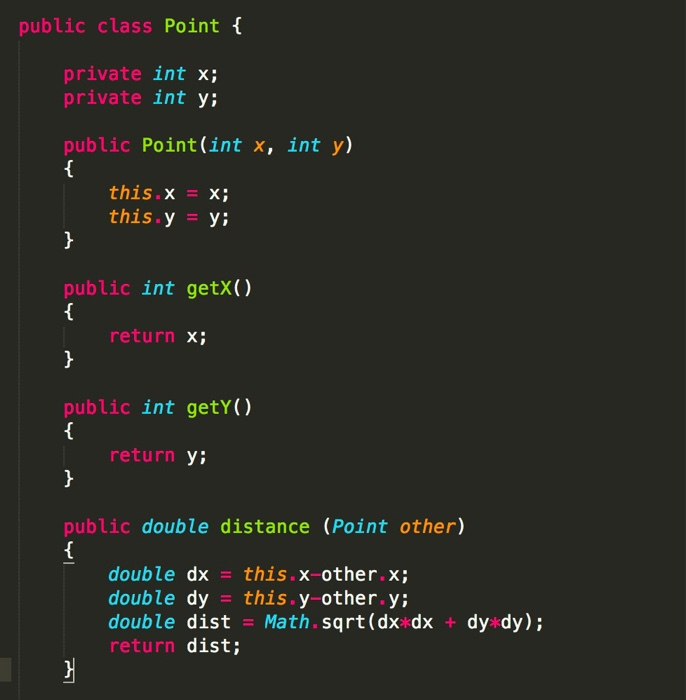public class Point f private int x; private int y; public Point(int x, int y) this.x X; this.y y; public int getX() return x; public int getY() return Y: public double distance (Point other) double dx this.x-other.x; double dy this.y-other.y double dist Math.sqrt(dx dx + dy dy); return dist;

• ### Question 11 (20 points) Given the class Point below, define a class Circle that represent a...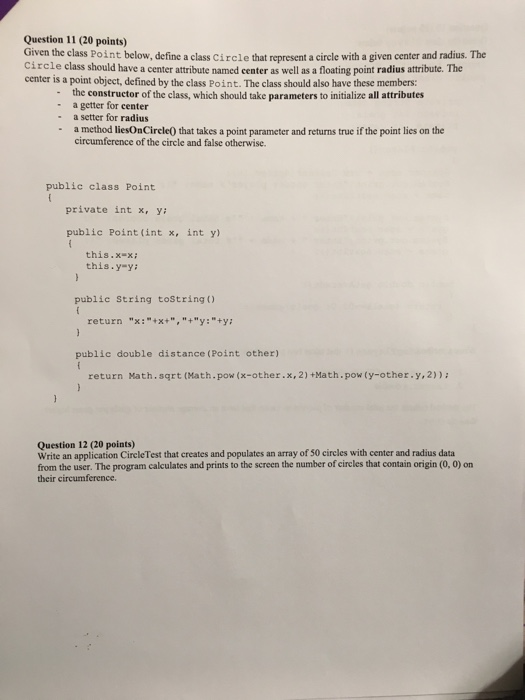Question 11 (20 points) Given the class Point below, define a class Circle that represent a circle with a given center and radius. The circle class should have a center attribute named center as well as a floating point radius attribute. The center is a point object, defined by the class Point. The class should also have these members: the constructor of the class, which should take parameters to initialize all attributes - a getter for center a setter for...

• ### What is wrong with the following code: class Point { private : int x, y; public...

What is wrong with the following code: class Point { private : int x, y; public : Point (int u, int v) : x(u), y(v) {} int getX () { return x; } int getY () { return y; } void setX (int newX ) const { x = newX ; } }; int main () { Point p(5, 3); p.setX (9001) ; cout << p. getX () << ’ ’ << p. getY (); return 0; }

• ### In Java Create a testing class that does the following to the given codes below: To...

In Java Create a testing class that does the following to the given codes below: To demonstrate polymorphism do the following: Create an arraylist to hold 4 base class objects Populate the arraylist with one object of each data type Code a loop that will process each element of the arraylist Call the first ‘common functionality’ method Call the second ‘common functionality’ method Call the third ‘common functionality’ method Verify that each of these method calls produces unique results Call...

• ### Consider the Automobile class: public class Automobile {    private String model;    private int rating;...

Consider the Automobile class: public class Automobile {    private String model;    private int rating; // a number 1, 2, 3, 4, 5    private boolean isTruck;    public Automobile(String model, boolean isTruck) {       this.model = model;       this.rating = 0; // unrated       this.isTruck = isTruck;    }        public Automobile(String model, int rating, boolean isTruck) {       this.model = model;       this.rating = rating;       this.isTruck = isTruck;    }                public String getModel()...

• ### Implement the Point class (code for this up to the isHigher method was discussed in the...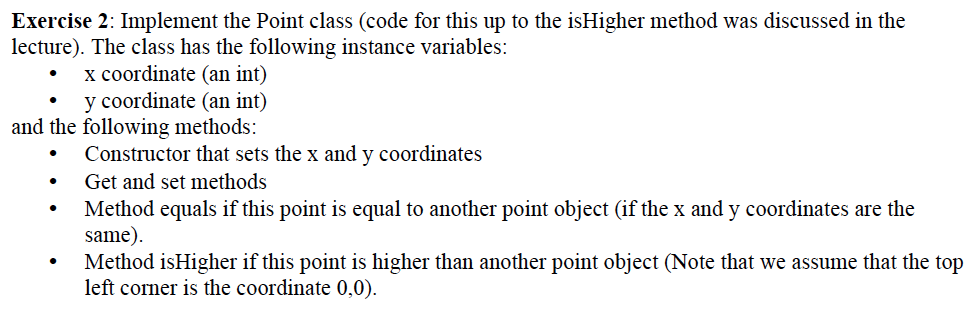Implement the Point class (code for this up to the isHigher method was discussed in the lecture). The class has the following instance variables: • x coordinate (an int) • y coordinate (an int) and the following methods: • Constructor that sets the x and y coordinates • Get and set methods • Method equals if this point is equal to another point object (if the x and y coordinates are the same). • Method isHigher if this point is...

• ### For this question you must write a java class called Rectangle and a client class called...

For this question you must write a java class called Rectangle and a client class called RectangleClient. The partial Rectangle class is given below. (For this assignment, you will have to submit 2 .java files: one for the Rectangle class and the other one for the RectangleClient class and 2 .class files associated with these .java files. So in total you will be submitting 4 files for part b of this assignment.) // A Rectangle stores an (x, y) coordinate...

• ### public class Fish { private String species; private int size; private boolean hungry; public Fish() {...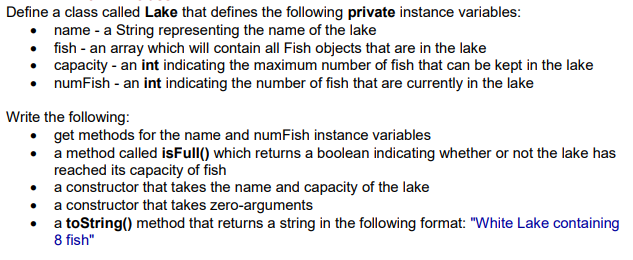public class Fish { private String species; private int size; private boolean hungry; public Fish() { } public Fish(String species, int size) { this.species = species; this.size = size; } public String getSpecies() { return species; } public int getSize() { return size; } public boolean isHungry() { return hungry; } public void setHungry(boolean hungry) { this.hungry = hungry; } public String toString() { return "A "+(hungry?"hungry":"full")+" "+size+"cm "+species; } }Define a class called Lake that defines the following private...

• ### Question 19 Given the following class: public class Swapper ( private int x; private String y...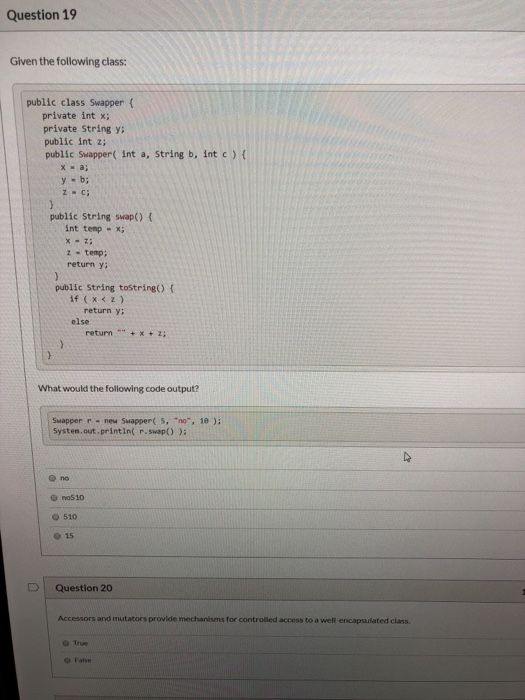Question 19 Given the following class: public class Swapper ( private int x; private String y public int z; public Swapper( int a, String b, int c) ( x-a; y b; zC; public String swap() ( int temp -x; x-z z temp; return y: public String tostring() ( if (x<z) return y: else return" +x+z What would the following code output? Swapper r new Suapper( 5, "no", 10); System.out.printin( r. swap ) ): no no510 510 e 15 Question 20...